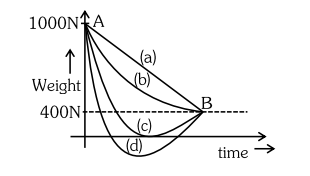# A person whose mass is 100 kg travels from Earth to Mars in a spaceship.Question:

A person whose mass is $100 \mathrm{~kg}$ travels from Earth to Mars in a spaceship. Neglect all other objects in sky and take acceleration due to gravity on the surface of the Earth and Mars as $10 \mathrm{~m} / \mathrm{s}^{2}$ and $4 \mathrm{~m} / \mathrm{s}^{2}$ respectively. Identify from the below figures, the curve that fits best for the weight of the passenger as a function of time.1. (c)

2. (a)

3. (d)

4. (b)

Correct Option: 1

Solution:

At neutral point $g=0$ so graph $(C)$ is correct Hence option (1).